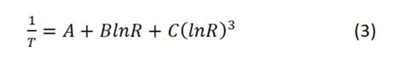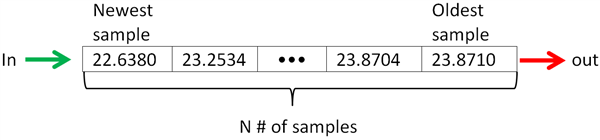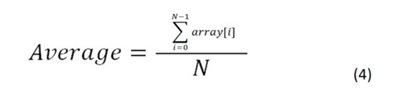# Designing with linear thermistors

The same hardware and software design methods commonly used with other positive temperature coefficient (PTC) or negative temperature coefficient (NTC) thermistors also apply to silicon-based linear thermistors. In this technical article, I’ll cover some simple hardware and software methods when using silicon-based linear thermistors.

Hardware

One method to generate an output voltage across a silicon-based linear thermistor is to use a voltage divider circuit, as shown in Figure 1. This results in a positive and linear voltage drop ( ) response, which is most often routed directly to the analog-to-digital converter (ADC).Figure 1: Low-side voltage divider biasing

The bias resistor value should be the resistance at 25°C (R25) of the silicon-based linear thermistor device in use. Table 1 shows the bias resistor values for several TI thermistors.

 Generic part number Rbias value TMP61 10 kΩ TMP63 100 kΩ TMP64 47 kΩ

Table 1: Bias resistor values

Alternatively, silicon-based linear thermistors can be biased directly using a precision current source, as shown in Figure 2. This method eliminates the need for a bias resistor and provides a greater dynamic voltage drop range.Figure 2: Precision current-source biasing

Keep in mind that while voltage and current sources are designed to be constant, they do vary slightly under different operating conditions, which could impact temperature measurement. Luckily, using a ratiometric approach can help negate the effect of power-supply variation because the ADC will read in a value proportional to its own reference voltage. As Figure 3 illustrates, it is possible to tie to the ADC reference voltage when using the voltage divider circuit.Figure 3: Ratiometric thermistor circuit

Software

Software for TI’s silicon-based linear thermistors is very simple, and our Thermistor Design Tool makes it even easier by providing resistance vs. temperature (R-T) tables, multiple temperature conversion methods and example code. When using the tool, make sure to select your device and enter your specific circuit parameters.

If you’re using a look-up table (LUT), you’ll find all of the resistance values across temperature under the Device Resistance Tables tab provided both in 1°C and 5°C steps. You can use the Lookup Table or Interpolation From Lookup Table tabs in the tool to get started with TI’s example code. The standard LUT method will round up or down to the nearest value found in the LUT.

The interpolation method in Equation 1 will calculate the temperature in between values found in the LUT for greater precision. Silicon-based linear thermistors can take advantage of interpolation because of their very stable, linear R-T response across temperature.

where X is the measured resistance, Y is the unknown value of temperature, X1 and Y1 are the lower-limit values in the LUT, and X2 and Y2 are the upper-limit values in the LUT.

As an alternative to LUTs, TI recommends using a curve-fit equation known as a fourth-order polynomial regression (Equation 2). Compared to other conversion methods, this equation (shown in Celsius) is very accurate and saves processing time on top of memory.You can find the solved coefficients for your design in the 4th Order Polynomial Regression tab of the Thermistor Design Tool. The tool also provides example code for the Steinhart-Hart equation (Equation 3, shown in Kelvin), a typical method of temperature conversion for thermistors:The Threshold Detection tab shows you what values you may expect to see from your ADC at specific temperatures for simple threshold monitoring. This method is helpful for applications where continuous temperature collection is unnecessary, but it’s critical for the system to stay above or below a temperature threshold.

Additionally, the Averaging tab explains how averaging your temperature samples can help increase the measurement resolution and signal-to-noise ratio. Using a first-in-first-out sequence, as shown in Figure 4, you can create a running average that calculates the temperature every time a new sample comes into the array.Figure 4: FIFO operation

When calculating the average, you would take the sum of all the values in your array and divide by the total number of samples (Equation 4).Switching to a silicon-based linear thermistor does not require much work, especially if you are already using another type of thermistor. A change required for any type of new thermistor would be in software. Easy to use and very versatile, TI’s linear thermistors come with all of the data and example codes you need, and our team of engineers on the TI E2E™ support forums can help you quickly set up your design. Regardless of your hardware or software preferences, our design tools help you get to market quickly and effectively.